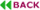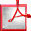doi: 10.7873/DATE.2015.0008

# Initial Transient Response of Oscillators with Long Settling Time

## ABSTRACT

The initial transient response of oscillators with high quality factor Q such as quartz crystal oscillators is orders of magnitudes larger than the period of oscillation. Therefore numerical solution by standard techniques of the underlying system of ordinary differential algebraic equations (DAEs) resulting from Kirchhoff’s current and voltage laws is run time inefficient. In this paper numerical techniques for the calculation of the initial transient response and steady state solution are investigated. The efficiency results from reformulating the underlying system of ordinary DAEs by a suitable system of partial DAEs, known as multirate PDE, and from suitable finite difference time domain (FDTD) methods with small numerical dissipation of energy. Unlike Harmonic Balance the waveforms are free of spurious oscillations, caused by the non-compactness of the trigonometric polynomials.

Keywords: Oscillator simulation, Quartz crystal oscillators, Initial transient response and steady state, Multirate PDE method, Trigonometric BDF methods, Optimal estimation of instantaneous frequency, Hilbert transformation.Full Text (PDF)